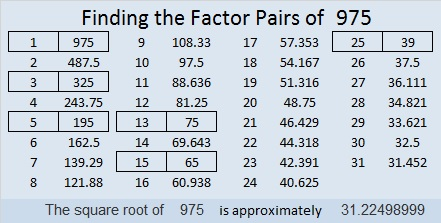# 975 and Level 5

This puzzle has eleven clues, some of which are designed to trick you possibly. Will you be tricked, or will you use logic to figure out where to put the factors from 1 to 10 in the first column and the top row? The finished puzzle looks like a multiplication table but with the factors out of numerical order.Print the puzzles or type the solution in this excel file: 10-factors-968-977

Here are a few facts about the number 975:

975 is the hypotenuse of SEVEN Pythagorean triples. Some factor pairs of 975 are highlighted in red next to those triples.
108-969-975 which is 3 times (36-323-325)
240-945-975 which is 15 times (16-63-65)
273-936-975 which is (7-24-25) times 39
375-900-975 which is (5-12-13) times 75
495-840-975 which is 15 times (33-56-65)
585-780-975 which is (3-4-5) times 195
612-759-975 which is 3 times (204-253-325)

1(5) + 2(5³) + 3(5²) + 4(5¹) + 5(5) = 975

You might think that last fact means that 975 is 12345 in base 5, but it isn’t. The only digits used in base 5 are 0, 1, 2, 3, and 4.

Here is 975 written in some different bases:
1111001111 in BASE 2 because 2⁹ + 2⁸ + 2⁷+ 2⁶ + 2³ + 2² + 2¹ + 2⁰ = 975
33033 in BASE 4 because 3(4⁴) + 3(4³) + 0(4²) + 3(4¹) + 3(4⁰) = 3(256 + 64 + 4 + 1) = 3(325) = 975
1717 in BASE 8 because 1(8³) + 7(8²) + 1(8¹) + 7(8⁰) = 975
303 in BASE 18 because 3(18²) + 0(18¹) + 3(18⁰) = 3(324 + 1) = 3(325) = 975

• 975 is a composite number.
• Prime factorization: 975 = 3 × 5 × 5 × 13, which can be written 975 = 3 × 5² × 13
• The exponents in the prime factorization are 1, 2, and 1. Adding one to each and multiplying we get (1 + 1)(2 + 1)(1 + 1) = 2 × 3 × 2 = 12. Therefore 975 has exactly 12 factors.
• Factors of 975: 1, 3, 5, 13, 15, 25, 39, 65, 75, 195, 325, 975
• Factor pairs: 975 = 1 × 975, 3 × 325, 5 × 195, 13 × 75, 15 × 65, or 25 × 39,
• Taking the factor pair with the largest square number factor, we get √975 = (√25)(√39) = 5√39 ≈ 31.22499This site uses Akismet to reduce spam. Learn how your comment data is processed.